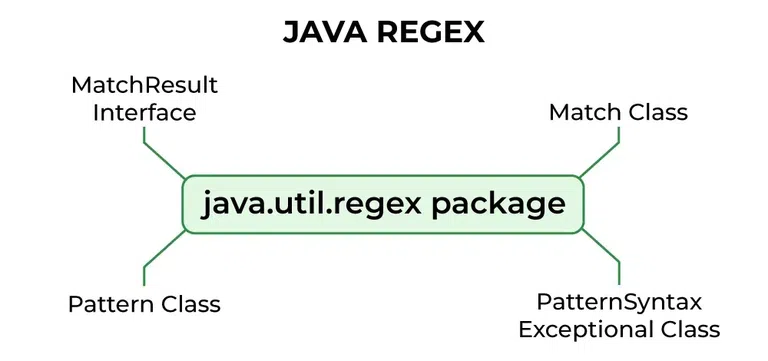GeeksforGeeks App
Open AppBrowser
Continue

## Related Articles

• Write an Interview Experience
• Java Tutorial
• Java Collection Tutorial

# Regular Expressions in Java

In Java, Regular Expressions or Regex (in short) in Java is an API for defining String patterns that can be used for searching, manipulating, and editing a string in Java. Email validation and passwords are a few areas of strings where Regex is widely used to define the constraints. Regular Expressions in Java are provided under java.util.regex package. This consists of 3 classes and 1 interface. The java.util.regex package primarily consists of the following three classes as depicted below in tabular format as follows:

## Regex Classes and Interfaces

Regex in Java provides 3 classes and 1 interface which are as follows:

More understanding can be interpreted from the image provided below as follows:## Pattern Class

This class is a compilation of regular expressions that can be used to define various types of patterns, providing no public constructors. This can be created by invoking the compile() method which accepts a regular expression as the first argument, thus returning a pattern after execution.

Example: Pattern class

## Java

 `// Java Program Demonstrating Working of matches() Method``// Pattern class` `// Importing Pattern class from java.util.regex package``import` `java.util.regex.Pattern;` `// Main class``class` `GFG {` `    ``// Main driver method``    ``public` `static` `void` `main(String args[])``    ``{` `        ``// Following line prints "true" because the whole``        ``// text "geeksforgeeks" matches pattern``        ``// "geeksforge*ks"``        ``System.out.println(Pattern.matches(``            ``"geeksforge*ks"``, ``"geeksforgeeks"``));` `        ``// Following line prints "false" because the whole``        ``// text "geeksfor" doesn't match pattern "g*geeks*"``        ``System.out.println(``            ``Pattern.matches(``"g*geeks*"``, ``"geeksfor"``));``    ``}``}`

Output

```true
false```

## Matcher class

This object is used to perform match operations for an input string in Java, thus interpreting the previously explained patterns. This too defines no public constructors. This can be implemented by invoking a matcher() on any pattern object.

Note: T Pattern.matches() checks if the whole text matches with a pattern or not. Other methods (demonstrated below) are mainly used to find multiple occurrences of patterns in the text.

Let us do discuss a few sample programs as we did for the Pattern class. Here we will be discussing a few Java programs that demonstrate the workings of compile(), find(), start(), end(), and split() in order to get a better understanding of the Matcher class.

Example 1: Pattern Searching

## Java

 `// Java program to demonstrate working of``// String matching in Java` `// Importing Matcher and Pattern class``import` `java.util.regex.Matcher;``import` `java.util.regex.Pattern;` `// Main class``class` `GFG {` `    ``// Main driver method``    ``public` `static` `void` `main(String args[])``    ``{` `        ``// Create a pattern to be searched``        ``// Custom pattern``        ``Pattern pattern = Pattern.compile(``"geeks"``);` `        ``// Search above pattern in "geeksforgeeks.org"``        ``Matcher m = pattern.matcher(``"geeksforgeeks.org"``);` `        ``// Finding string using find() method``        ``while` `(m.find())` `            ``// Print starting and ending indexes``            ``// of the pattern in the text``            ``// using this functionality of this class``            ``System.out.println(``"Pattern found from "``                               ``+ m.start() + ``" to "``                               ``+ (m.end() - ``1``));``    ``}``}`

Output

```Pattern found from 0 to 4
Pattern found from 8 to 12```

## Regex Character classes

Below is the implementation of the above topic:

## Java

 `// Java Program to check on Regex``import` `java.io.*;``import` `java.util.regex.*;` `// Driver class``class` `GFG {``    ``// Main function``    ``public` `static` `void` `main(String[] args)``    ``{``        ``// Checks if the string matches with the regex``        ``// Should be single character a to z``        ``System.out.println(Pattern.matches(``"[a-z]"``, ``"g"``));` `        ``// Check if the element is range a to z or A to Z``        ``System.out.println(``            ``Pattern.matches(``"[a-zA-Z]"``, ``"Gfg"``));``    ``}``}`

Output

```true
false```

## Regex Metacharacters

Below is the implementation of Regex Metacharacters:

## Java

 `// Java Program to check on regex``import` `java.io.*;``import` `java.util.regex.*;` `// Driver class``class` `GFG {``    ``// Main function``    ``public` `static` `void` `main(String[] args)``    ``{``        ``// Checking all the strings using regex``        ``System.out.println(Pattern.matches(``"[b-z]?"``, ``"a"``));` `        ``// Check if all the elements are in range a to z``        ``// or A to Z``        ``System.out.println(``            ``Pattern.matches(``"[a-zA-Z]+"``, ``"GfgTestCase"``));` `        ``// Check if elements is not in range a to z``        ``System.out.println(Pattern.matches(``"[^a-z]?"``, ``"g"``));` `        ``// Check if all the elements are either g,e,k or s``        ``System.out.println(``            ``Pattern.matches(``"[geks]*"``, ``"geeksgeeks"``));``    ``}``}`

Output

```false
true
false
true```

## Java Regex Finder Example

Below is the implementation of the Java Regex Finder:

## Java

 `// Java Program to implement regex``import` `java.io.*;``import` `java.util.regex.*;` `// Driver Class``class` `GFG {``    ``// Main Function``    ``public` `static` `void` `main(String[] args)``    ``{``        ``// Check if all elements are numbers``        ``System.out.println(Pattern.matches(``"\\d+"``, ``"1234"``));` `        ``// Check if all elements are non-numbers``        ``System.out.println(Pattern.matches(``"\\D+"``, ``"1234"``));` `        ``// Check if all the elements are non-numbers``        ``System.out.println(Pattern.matches(``"\\D+"``, ``"Gfg"``));` `        ``// Check if all the elements are non-spaces``        ``System.out.println(Pattern.matches(``"\\S+"``, ``"gfg"``));``    ``}``}`

Output

```true
false
true
true```

## Conclusion

Lastly, let us do discuss some of the important observations as retrieved from the above article

1. We create a pattern object by calling Pattern.compile(), there is no constructor. compile() is a static method in the Pattern class.
2. Like above, we create a Matcher object using matcher() on objects of the Pattern class.
3. Pattern.matches() is also a static method that is used to check if a given text as a whole matches the pattern or not.
4. find() is used to find multiple occurrences of patterns in the text.
5. We can split a text based on a delimiter pattern using the split() method

## FAQs in Java Regex

### Q1. What are regular expressions in Java?

Ans:

Regular Expressions in java are used for string patterns that can be used for searching, manipulating, and editing a string in Java.

### Q2. What is a simple example of regular expression in Java?

Ans:

A simple example of a regular expression in java is mentioned below:

## Java

 `// Java Program to check on Regex``import` `java.io.*;``import` `java.util.regex.*;` `// Driver class``class` `GFG {``    ``// Main function``    ``public` `static` `void` `main(String[] args)``    ``{``        ``// Checks if the string matches with the regex``        ``// Should be single character a to z``        ``System.out.println(Pattern.matches(``"[a-z]"``, ``"g"``));` `         ``// Check if all the elements are non-numbers``        ``System.out.println(Pattern.matches(``"\\D+"``, ``"Gfg"``));` `        ``// Check if all the elements are non-spaces``        ``System.out.println(Pattern.matches(``"\\S+"``, ``"gfg"``));``    ``}``}`

Output

```true
true
true```